Open in App
Not now

# Bar chart using Plotly in Python

• Last Updated : 08 Jul, 2021

Plotly is a Python library which is used to design graphs, especially interactive graphs. It can plot various graphs and charts like histogram, barplot, boxplot, spreadplot, and many more. It is mainly used in data analysis as well as financial analysis. Plotly is an interactive visualization library.

## Bar Chart

In a bar chart the data categories are displayed on the vertical axis and the data values are displayed on the horizontal axis. Labels are easier to display and with a big data set they impel to work better in a narrow layout such as mobile view.

Syntax: plotly.express.bar(data_frame=None, x=None, y=None, color=None, facet_row=None, facet_col=None, facet_col_wrap=0, hover_name=None, hover_data=None, custom_data=None, text=None, error_x=None, error_x_minus=None, error_y=None, error_y_minus=None, animation_frame=None, animation_group=None, category_orders={}, labels={}, color_discrete_sequence=None, color_discrete_map={}, color_continuous_scale=None, range_color=None, color_continuous_midpoint=None, opacity=None, orientation=None, barmode=’relative’, log_x=False, log_y=False, range_x=None, range_y=None, title=None, template=None, width=None, height=None)

Parameters:

### Bar chart with Plotly Express

In this example, we are going to use Plotly express to plot a bar chart.

## Python3

 `import` `plotly.express as px``import` `numpy` `# creating random data through randomint``# function of numpy.random``np.random.seed(``42``)``  ` `random_x``=` `np.random.randint(``1``, ``101``, ``100``)``random_y``=` `np.random.randint(``1``, ``101``, ``100``)` `fig ``=` `px.bar(random_x, y ``=` `random_y)``fig.show()`

Output: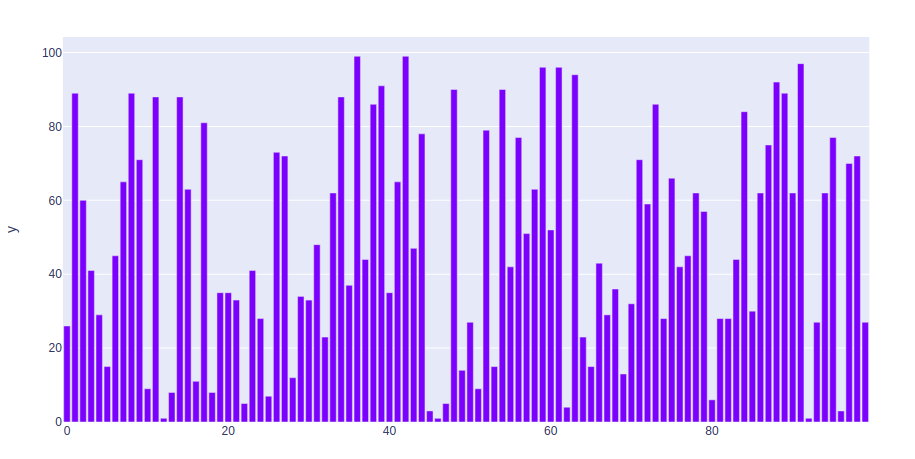### Bar chart with Long and wide Format Data

Example 1: In this example, we will use the iris data set and convert it into the dataframe to plot bar chart.

## Python3

 `import` `plotly.express as px` `df ``=` `px.data.iris()` `fig ``=` `px.bar(df, x ``=` `"sepal_width"``, y ``=` `"sepal_length"``)``fig.show()`

Output: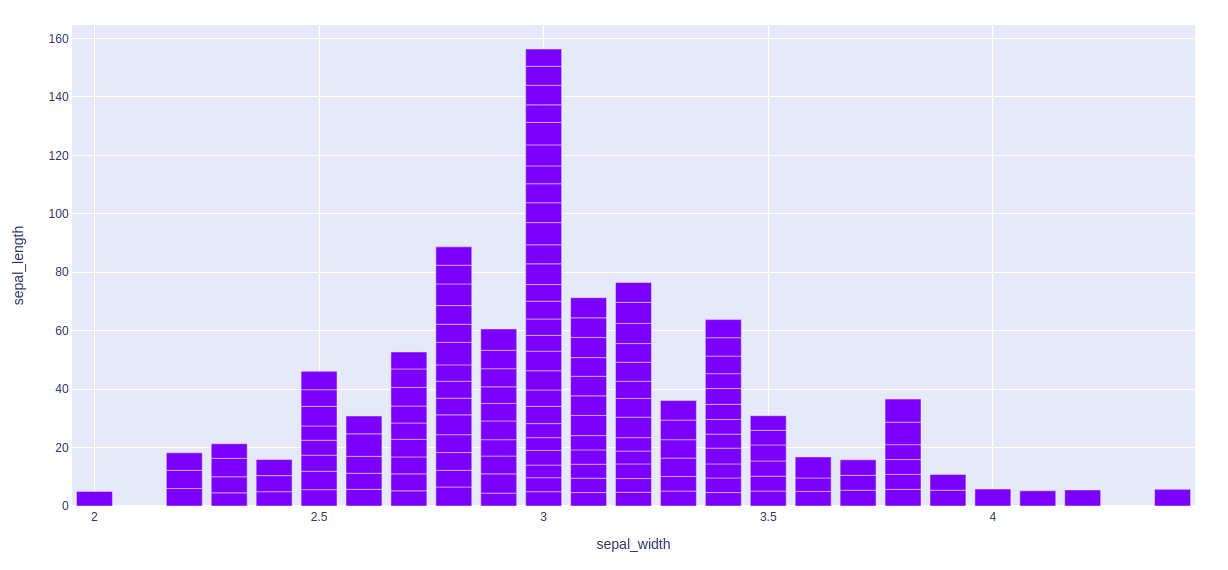Example 2: In this example, we will see long-form data. long-form data has one row per observation, and one column per variable. This is suitable for storing and displaying multivariate data i.e. with dimensions greater than 2. This format is sometimes called “tidy”.

## Python3

 `import` `plotly.express as px``long_df ``=` `px.data.medals_long()` `fig ``=` `px.bar(long_df, x ``=` `"nation"``, y ``=` `"count"``,``             ``color ``=` `"medal"``, title ``=` `"Long-Form Input"``)``fig.show()`

Output: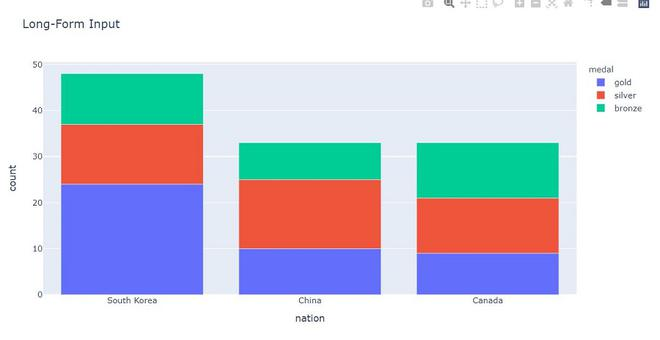Example 3: In this example, we will see wide-from data. wide-form data has one row per value of one of the first variable, and one column per value of the second variable. This is suitable for storing and displaying 2-dimensional data.

## Python3

 `import` `plotly.express as px``df ``=` `px.data.medals_wide()` `fig ``=` `px.bar(df, x``=``"nation"``,``             ``y``=``[``"gold"``, ``"silver"``, ``"bronze"``],``             ``title``=``"Wide Form Data"``)``fig.show()`

Output: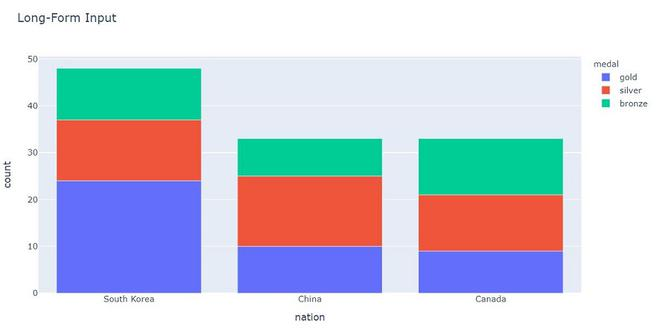## Showing Facetted subplots

In plotly, to create the facetted subplots facet_row argument is used, where different values correspond to different rows of the dataframe columns.

Example:

## Python3

 `import` `plotly.express as px` `df ``=` `px.data.iris()` `fig ``=` `px.bar(df, x``=``"sepal_width"``, y``=``"sepal_length"``,``             ``color``=``"species"``, barmode``=``"group"``,``             ``facet_row``=``"species"``, facet_col``=``"species_id"``)` `fig.show()`

Output: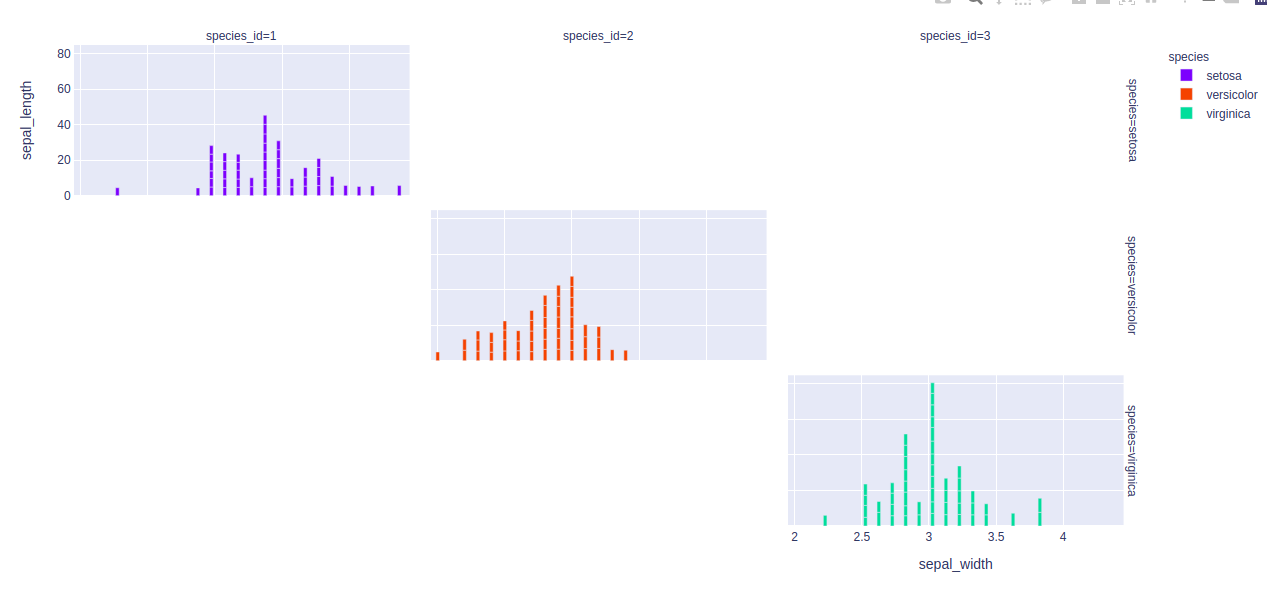## Customizing bar charts

By using keyword arguments the bar mode can be customized. Let’s see the examples given below:

Example 1: In this example, the bar is customizing according to the color attributes.

## Python3

 `import` `plotly.express as px` `df ``=` `px.data.iris()` `fig ``=` `px.bar(df, x``=``"sepal_width"``, y``=``"sepal_length"``, color``=``"species"``)``fig.show()`

Output: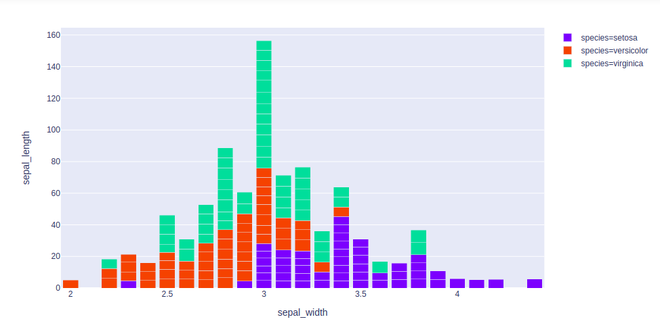Example 2: In this example, we will use barmode = “group”. With “group”, the bars are grouped with one another

## Python3

 `import` `plotly.express as px` `df ``=` `px.data.iris()` `fig ``=` `px.bar(df, x``=``"sepal_width"``, y``=``"sepal_length"``,``             ``color``=``"species"``, barmode ``=` `'group'``)``fig.show()`

Output: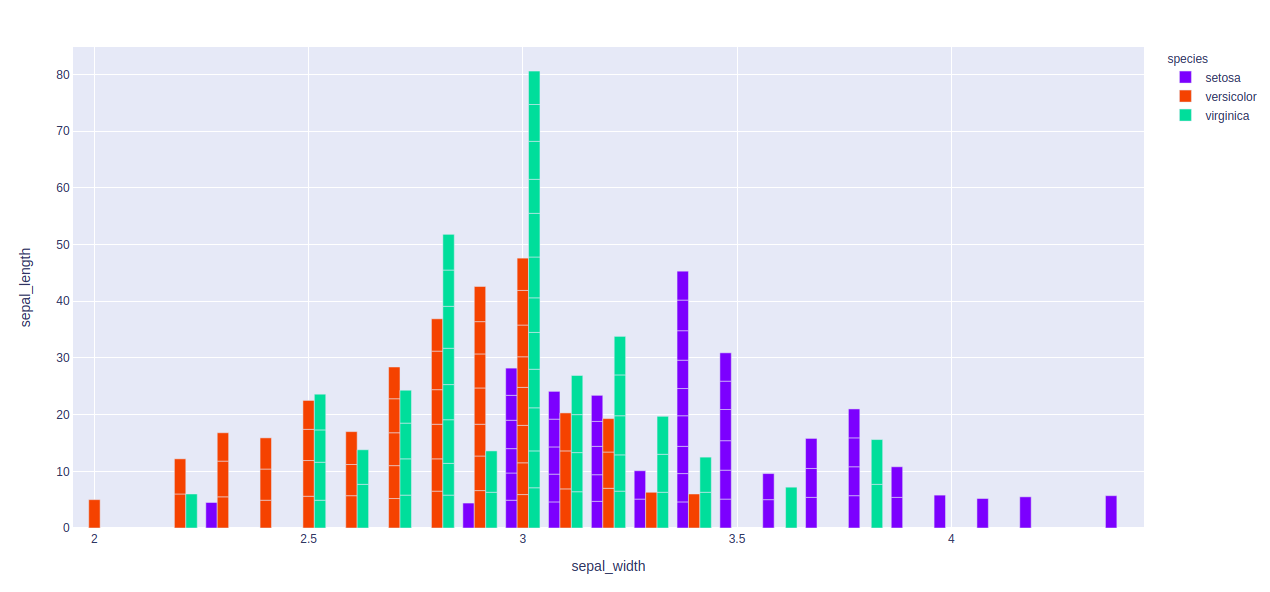Example 3: Here, we will use “overlay” With “overlay”, the bars are overlaid with another. Here we see easily “opacity” to see multiple bars.

## Python3

 `import` `plotly.express as px` `df ``=` `px.data.iris()` `fig ``=` `px.bar(df, x``=``"sepal_width"``, y``=``"sepal_length"``,``             ``color``=``"species"``, barmode``=``'overlay'``)``fig.show()`

Output: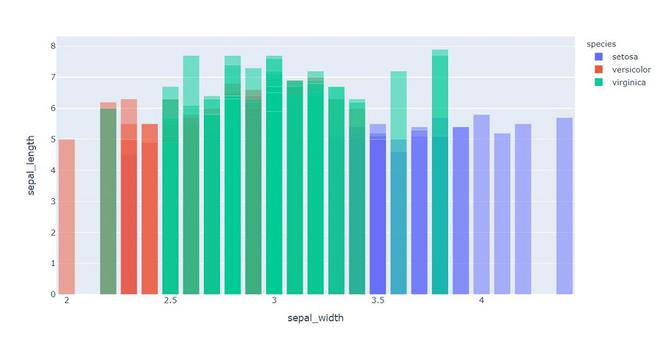My Personal Notes arrow_drop_up